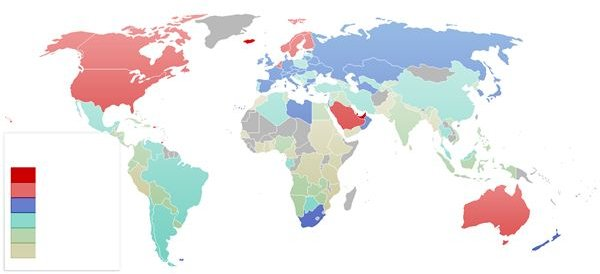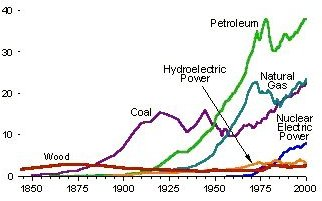# Where Does US Energy Come From?

## Where does the World’s Energy come From?

The world’s total energy consumption in 2008 was 474 exajoules (474×1018 J).

Total energy consumption in 1980 was 9.48 TW (terrawatts – a terawatt is equals to 1012 watts), and it increased to 15.8 TW in 2006. Most of the world’s energy comes from oil, gas, and coal. Oil, gas, and coal consumption in 2006 was 5.74 TW, 3.61 TW, and 4.27 TW respectively. Therefore 86.2% of total of the world’s energy consumption is fossil fuels.Hydropower and nuclear power consumption was 1.00 TW and 0.93 TW respectively in 2006 and their percentage contribution was only 12.3%. Other energy sources such as geothermal, wind, solar energy, and wood contributed only 0.16 TW, but they show a significant increase compared to 1980. For a comparison, although the total power used by humans in 2006 was 16 TW, the average peak power output of a single lightning strike is about 1 TW.

## Where does US Energy come From?If you ask where US energy comes from, the answer is that there is not much difference between the US and the world’s energy consumption behavior as the US represents 25% of the world’s energy consumption. Petroleum is the primary energy source in the US. Total US energy consumption in 2009 was 94.6 quadrillion BTUs (quads) according to the Annual energy review of U.S. Energy Information Administration. One Btu or British thermal unit equals to about 1,055.05585 joules and a quadrillion equals to 1015. The estimated U.S. energy use in 2008 was 99.2 quadrillion BTUs and it has been decreased in 2009.

Petroleum accounts for 35.3 quadrillion BTUs (quads) which is a 37.3% of total energy supply. Natural gas and coal energy supply was 23.4 quads and 19.7 quads respectively. The respective percentages are 24.7% and 20.8%. The contribution of renewable energy and nuclear power was 7.7 quads and 8.3 quads respectively.

There was a sharp increase of renewable energy in 2009- by 0.19 quads- compared to 2008. This is mainly due to incentives and technological developments in the sector. With the frequent fluctuations of oil prices and supply, there’s an additional motivation among many nations for non-fossil fuel based energy sources in the world. Therefore the renewable energy sector has been gaining a remarkable increase during the past several years. Nuclear energy use remained relatively flat in 2009, and the use of coal significantly decreased due to lower electricity demand and a fuel shift to natural gas.

## Energy Demand SectorsMost of the US energy that comes from coal and nuclear energy sources was used for electric power generation. 100% of nuclear power and 93% of coal was used for the production of electric power in 2009 and the electric energy generated was 38.3 quads. 94% of energy that come from petroleum was used for the transportation sector and the energy consumption was 27 quads in 2009. The main energy source for the residential and commercial sector was natural gas and the percentage contribution was 76%. The industrial sector that consumes 18.8 quads used petroleum and natural gas as its main energy sources with respective percentages of 41% and 40%.

With the environmental considerations, there’s an increase of renewables in USA. Also there’s a decrease of total energy consumption during year 2009 compared to 2008. Part of that decrease was due to technological advancements and increase of energy efficiency (and some of it was due to a continued economic recession). With the scarcity of fossil energy resources, we can expect that the word’s energy that comes from various sources will follow what happens in the US.